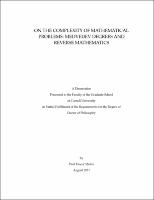## On The Complexity Of Mathematical Problems: Medvedev Degrees And Reverse Mathematics##### Abstract
We investigate the complexity of mathematical problems from two perspectives: Medvedev degrees and reverse mathematics. In the Medvedev degrees, we calculate the complexity of its first-order theory, and we also calculate the complexities of the first-order theories of several related structures. We characterize the join-irreducible Medvedev degrees and deduce several consequences for the interpretation of propositional logic in the Medvedev degrees. We equate the size of chains of Medvedev degrees with the size of chains of sets of reals under ⊆. In reverse mathematics, we analyze the strength of classical theorems of finite graph theory generalized to the countable. In particular, we consider Menger's theorem, Birkhoff's theorem, and unfriendly partitions.
2011-08-31
##### Keywords
mass problems; Medvedev degrees; reverse mathematics
Shore, Richard A
##### Committee Member
Nerode, Anil
Kozen, Dexter Campbell
Mathematics
##### Degree Name
Ph. D., Mathematics
##### Degree Level
Doctor of Philosophy
##### Types
dissertation or thesis